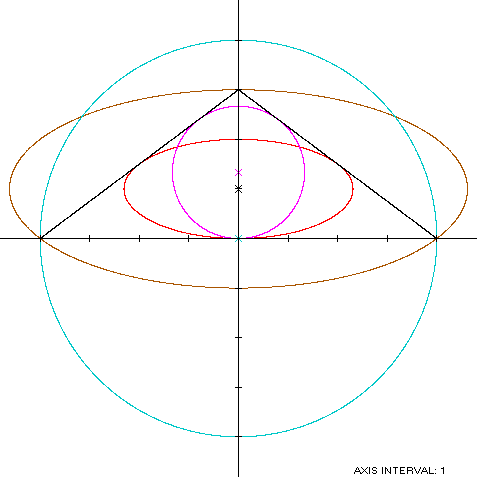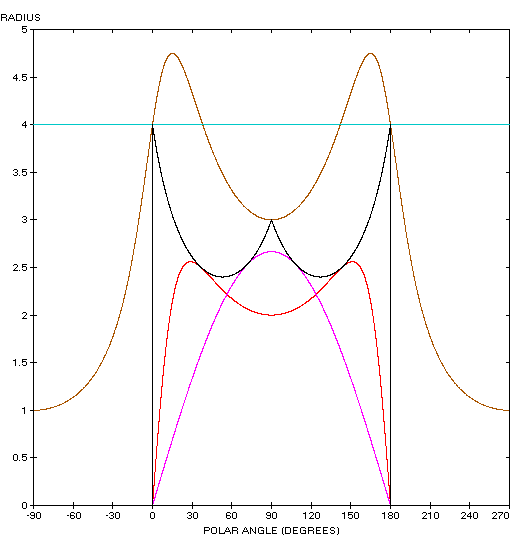Isosceles Triangle
ISOSCELES TRIANGLE

Balmoral Software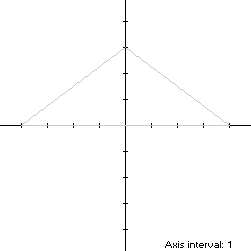Consider a y-symmetric isosceles triangle with vertices (0,v) and (±u,0), u,v > 0. The width x height of its bounding rectangle is 2u x v.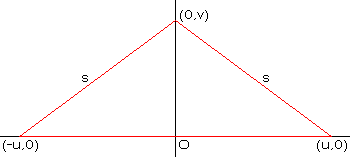Let t represent the usual parameter, ranging from 0 to 2π through the quadrants in counterclockwise order. The parametric equations of the triangle are piecewise linear in t: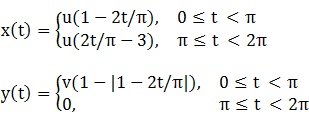### Metrics

The lateral side length of the triangle is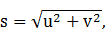so its perimeter is 2(u + s) and its area is uv. Its centroid is the average of its vertex coordinates: (0,v/3).

### Incircle

The radius and center ordinate of the triangle's largest bounded circle (its incircle) are
R = d = u(s - u)/v

### Inellipse

Using z = 0 in Lemma E,
d/dt x(t)[y(t) - z] = d/dt uv(1 - 2t/π)(1 - |1 - 2t/π|)
has a zero over [0,π) at t* = π/4. The corresponding coordinates are:
x* = u/2

y* = v/2,

so the inellipse dimensions are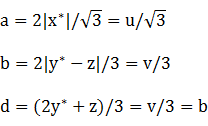For verification,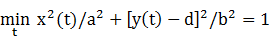### Circumellipse

Using h = v and w = 2u in Lemma TE, the parameters of the circumellipse are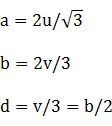### Circumcircle

If the triangle is obtuse (u > v), its apex point (0,v) is not tangent to its bounding circle. In that case, the triangle's smallest bounding circle has a diameter between the vertices (±u,0) and its center at the origin.

If the triangle is acute (u ≤ v), then using h = v and w = 2u in Lemma TC, the circumellipse has radius and center ordinate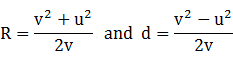### Summary Table

 Perimeter Area Centroid Figure Parameters Incircle R = u(s - u)/v 2πR πR2 (0,R) Inellipse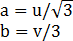Computed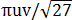(0,v/3) Isosceles triangle Width: 2uHeight: v 2(u + s) uv (0,v/3) Circumellipse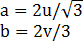Computed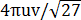(0,v/3) Bounding circle Obtuse: R = u 2πu πu2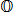Acute: R =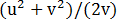2πR πR2 (0,v - R)
The centers of the boundary ellipses are both the same as the centroid of the isosceles triangle, and the circumellipse is exactly twice as large as the inellipse.

Specific Example

The boundary conics for an obtuse isosceles triangle with u = 4 and v = 3 are shown below: